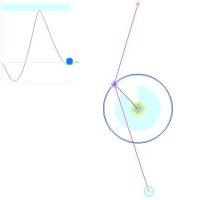# Zeeman

## Exhibit: table of content

##### Oliver Knill, SciCtr 434, knill.harvard.eduMove with the mouse the red marker and watch what happens. Applet information: Based on an applet of L. Griebl. Modifications (mostly eyecandy and change of geometry) by Oliver Knill, 2000. An angle sector visualization was added in July 2005. The Zeeman catastrophe machine illustrates that discontinuities can occur naturally also in completely smooth setups. The mouse position (x,y) determines the wheel angle z=f(x,y). The graph to the left shows the potential energy V(x,y,z) depending on the mouse pointer (x,y) and the angle z. The function z=f(x,y) is determined by the property that the partial derivative g(x,y,z) = Vz(x,y,z) is zero. The interpretation is that the wheel angle z settels so that the potential energy is a local minimum. For fixed (x,y), the function h(z) = g(x,y,z) has different minima in general as you can see when watching the graph of h in the applet. The value f(x,y) choses a local minimal energy configuration. But if a local minimum disappears, the next local minimum can be far away and the angle can jump. Again: while the angle z is always on the smooth surface g(x,y,z) = 0, the function f(x,y) is discontinuous. Discontinuities are also called catastrophes.